# Java Program to Find Distance Between 2 Points

In this tutorial you will learn about the Java Program to Find Distance Between 2 Points and its application with practical example.

In this tutorial, we will learn to create a Java Program to Find Distance between 2 point using Java programming.

## Prerequisites

Before starting with this tutorial we assume that you are best aware of the following Java programming topics:

• Java Operators.
• Basic Input and Output function in Java.
• Class and Object in Java.
• Basic Java programming.
• If-else statements in Java.
• For loop in Java.

## Distance Between two Points

Using Pythagorean theorem to find the distance between two points (x1, y1) and (x2, y2), all we need are the coordinates in pairs and apply the formula.

Distance Formula:

Distance:- √((x2 – x1)2 + (y2 -y1)2).

Assume :=> We have two points A and B, with coordinates (x1, y1) and (x2, y2) respectively. So their distance  can be represented as AB and it can be calculated as.

The first point (A):- (x1, y1)
Second point (B):- (x2, y2)

## Java Program to Find Distance Between 2 Points

In this our program we will create a program to find Distance Between two points. We would first declared and initialized the required variables. Next, we would prompt user to input value.Later in the program we will find Distance between points.

## Output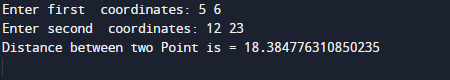In the above program, we have first declared and initialized a set variables required in the program.

• x1 = it will hold value of first coordinates
• y1 = it will hold value of first coordinates
• x2 = it will hold value of second coordinates
• y2 = it will hold value of second coordinates
• x= it will represent distance.
• y= it will represent distance.
• distance= it will display final result.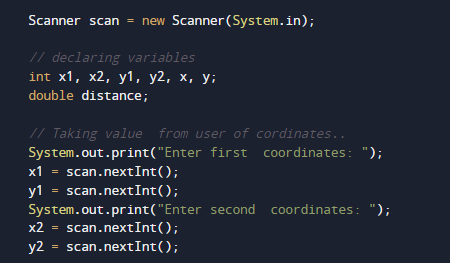the Formula to find the distance between two points is: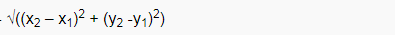To calculate the distance between two points “(x1,y1) and (x2,y2) or √ ( x )2+( y )2 . All you need is use the coordinates formula shown below.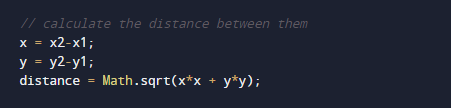Here we will to use following formula “ Math.sqrt(x*x+y*y))” and Math.sqrt() function which is define in Java standard library.it will take two parameter as a arguments x and y values supply to it.And in return gives the required output.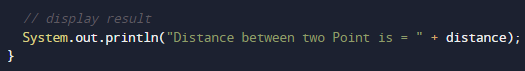As shown in image above.

In this tutorial we have learn about the Java Program to Find Distance Between 2 Points and its application with practical example. I hope you will like this tutorial.